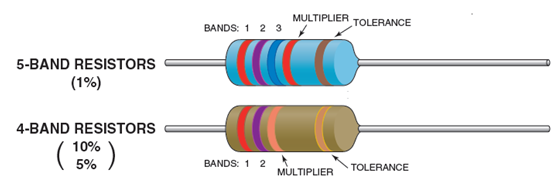### Resistors Colour Code

many resistors use coloured painted bands which represent a number and multiplier to indicate both their resistive value and their tolerance with the physical size of the resistor indicating its wattage rating. These coloured painted bands produce a system of identification generally known as a Resistors Colour Code.

There are two resistor colour coded systems, the four-band system and the five-band system, which use coloured bands or rings that completely encircle its body to identify the resistors value. These coloured bands are usually printed towards one end of the resistors body to indicate the first digit with the colours being read from left to right.four-band system
In the four-band system, the first band closest to the edge represents the first digit of the resistance value, the second band is the second digit, the third band is the decimal multiplier, which tells us how many zeros to add after the first two digits and the fourth band is the tolerance giving Digit, Digit, Multiplier, Tolerance. Resistors having standard tolerance value of 20% usually do not have a fourth band.

five-band system
The five-band system displays the coloured bands the same as for the four-band, except for an additional third coloured band to represent a third significant digit giving, Digit, Digit, Digit, Multiplier, Tolerance. The five-band system is used for high precision resistors with low tolerance.

Examples: the reading from left to right
example 1:first band red = 2    (digit)
second band red = 2   (digit)
third band black = x 1   (multiplier)
forth gold = 5%  (tolerance)
the value is = 22 x 1 = 22 Ohms  with tolerance 5%

example 2:brown (1)- black (0) - orange (x1000) - gold
10x1000 = 10 k ohms  with tolerance 5%

example 3:yellow - violet - orang - gold
47 k ohms  with tolerance 5%

example 4:first band red = 2    (digit)
second band red = 2   (digit)
third band black = 0   (digit)
forth bandbrown = x 10  (multiplier)
fifth band brwon = 1%    (tolerance)
the value is = 220 x 10 = 2.2K Ohms  with tolerance 1%

example 5:green - blue - black - orange - red
560 k ohm with tolerance 2%